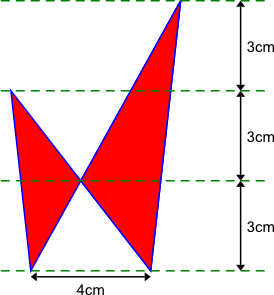#### You may also like### Isosceles

Prove that a triangle with sides of length 5, 5 and 6 has the same area as a triangle with sides of length 5, 5 and 8. Find other pairs of non-congruent isosceles triangles which have equal areas.### Trice

ABCDEFGH is a 3 by 3 by 3 cube. Point P is 1/3 along AB (that is AP : PB = 1 : 2), point Q is 1/3 along GH and point R is 1/3 along ED. What is the area of the triangle PQR?### Ratty

If you know the sizes of the angles marked with coloured dots in this diagram which angles can you find by calculation?

# Kissing Triangles

##### Age 11 to 14Challenge Level
Determine the total area of the two kissing triangles shaded red.Is there more than one way of drawing the shape?